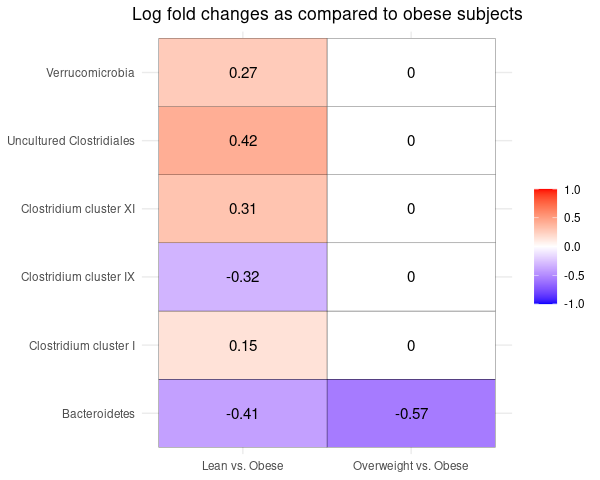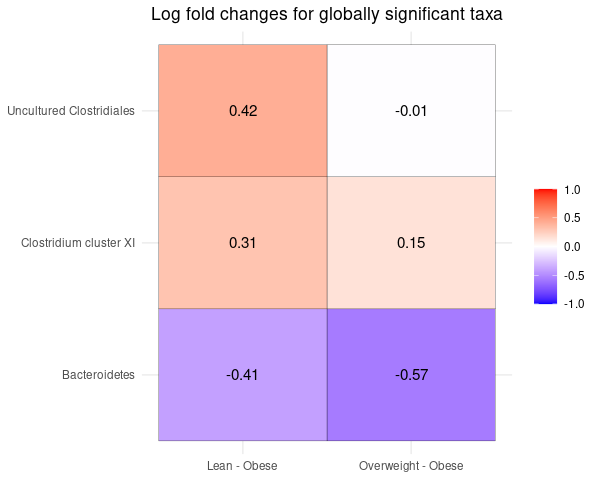# 1. Introduction

Analysis of Compositions of Microbiomes with Bias Correction (ANCOM-BC) (Lin and Peddada 2020) is a methodology of differential abundance (DA) analysis for microbial absolute abundances. ANCOM-BC estimates the unknown sampling fractions, corrects the bias induced by their differences through a log linear regression model including the estimated sampling fraction as an offset terms, and identifies taxa that are differentially abundant according to the variable of interest. For more details, please refer to the ANCOM-BC paper.

# 2. Installation

if (!requireNamespace("BiocManager", quietly = TRUE))
install.packages("BiocManager")
BiocManager::install("ANCOMBC")

library(ANCOMBC)

# 3. Example Data

The HITChip Atlas dataset contains genus-level microbiota profiling with HITChip for 1006 western adults with no reported health complications, reported in (Lahti et al. 2014). The dataset is also available via the microbiome R package (Lahti et al. 2017) in phyloseq (McMurdie and Holmes 2013) format. In this tutorial, we consider the following covariates:

• Continuous covariates: “age”

• Categorical covariates: “region”, “bmi”

• The group variable of interest: “bmi”

• Three groups: “lean”, “overweight”, “obese”

• The reference group: “obese”

data(atlas1006)

# Subset to baseline
tse = atlas1006[, atlas1006$time == 0] # Re-code the bmi group tse$bmi = recode(tse$bmi_group, obese = "obese", severeobese = "obese", morbidobese = "obese") # Subset to lean, overweight, and obese subjects tse = tse[, tse$bmi %in% c("lean", "overweight", "obese")]

# Note that by default, levels of a categorical variable in R are sorted
# alphabetically. In this case, the reference level for bmi will be
# lean. To manually change the reference level, for instance, setting obese
# as the reference level, use:
tse$bmi = factor(tse$bmi, levels = c("obese", "overweight", "lean"))
# You can verify the change by checking:
# levels(sample_data(tse)$bmi) # Create the region variable tse$region = recode(as.character(tse$nationality), Scandinavia = "NE", UKIE = "NE", SouthEurope = "SE", CentralEurope = "CE", EasternEurope = "EE", .missing = "unknown") # Discard "EE" as it contains only 1 subject # Discard subjects with missing values of region tse = tse[, ! tse$region %in% c("EE", "unknown")]

print(tse)
class: TreeSummarizedExperiment
dim: 130 873
assays(1): counts
Yersinia et rel.
rowData names(3): Phylum Family Genus
colnames(873): Sample-1 Sample-2 ... Sample-1005 Sample-1006
colData names(12): age sex ... bmi region
reducedDimNames(0):
mainExpName: NULL
altExpNames(0):
rowTree: NULL
colTree: NULL

# 4 ANCOM-BC Implementation

## 4.1 Run ancombc function

out = ancombc(data = tse, assay_name = "counts",
tax_level = "Family", phyloseq = NULL,
formula = "age + region + bmi",
p_adj_method = "holm", prv_cut = 0.10, lib_cut = 1000,
group = "bmi", struc_zero = TRUE, neg_lb = TRUE, tol = 1e-5,
max_iter = 100, conserve = TRUE, alpha = 0.05, global = TRUE,
n_cl = 1, verbose = TRUE)

res = out$res res_global = out$res_global

# ancombc also supports importing data in phyloseq format
# tse_alt = agglomerateByRank(tse, "Family")
# pseq = makePhyloseqFromTreeSummarizedExperiment(tse_alt)
# out = ancombc(data = NULL, assay_name = NULL,
#               tax_level = "Family", phyloseq = pseq,
#               formula = "age + region + bmi",
#               p_adj_method = "holm", prv_cut = 0.10, lib_cut = 1000,
#               group = "region", struc_zero = TRUE, neg_lb = TRUE, tol = 1e-5,
#               max_iter = 100, conserve = TRUE, alpha = 0.05, global = TRUE,
#               n_cl = 1, verbose = TRUE)

## 4.2 ANCOMBC primary result

Result from the ANCOM-BC log-linear model to determine taxa that are differentially abundant according to the covariate of interest. It contains: 1) log fold changes; 2) standard errors; 3) test statistics; 4) p-values; 5) adjusted p-values; 6) indicators whether the taxon is differentially abundant (TRUE) or not (FALSE).

tab_lfc = res$lfc col_name = c("Intercept", "Age", "NE - CE", "SE - CE", "US - CE", "Overweight - Obese", "Lean - Obese") colnames(tab_lfc) = col_name tab_lfc %>% datatable(caption = "Log Fold Changes from the Primary Result") %>% formatRound(col_name, digits = 2) ### SE tab_se = res$se
colnames(tab_se) = col_name
tab_se %>%
datatable(caption = "SEs from the Primary Result") %>%
formatRound(col_name, digits = 2)

tab_w = res$W colnames(tab_w) = col_name tab_w %>% datatable(caption = "Test Statistics from the Primary Result") %>% formatRound(col_name, digits = 2) ### P-values tab_p = res$p_val
colnames(tab_p) = col_name
tab_p %>%
datatable(caption = "P-values from the Primary Result") %>%
formatRound(col_name, digits = 2)

tab_q = res$q colnames(tab_q) = col_name tab_q %>% datatable(caption = "Adjusted p-values from the Primary Result") %>% formatRound(col_name, digits = 2) ### Differentially abundant taxa tab_diff = res$diff_abn
colnames(tab_diff) = col_name
tab_diff %>%
datatable(caption = "Differentially Abundant Taxa from the Primary Result")

### Bias-corrected abundances

Step 1: obtain estimated sample-specific sampling fractions (in log scale).

Step 2: correct the log observed abundances by subtracting the estimated sampling fraction from log observed abundances of each sample.

Note that we are only able to estimate sampling fractions up to an additive constant. Thus, only the difference between bias-corrected abundances are meaningful.

samp_frac = out$samp_frac # Replace NA with 0 samp_frac[is.na(samp_frac)] = 0 # Add pesudo-count (1) to avoid taking the log of 0 log_obs_abn = log(out$feature_table + 1)
# Adjust the log observed abundances
log_corr_abn = t(t(log_obs_abn) - samp_frac)
# Show the first 6 samples
round(log_corr_abn[, 1:6], 2) %>%
datatable(caption = "Bias-corrected log observed abundances")

### Visualization for age

df_lfc = data.frame(res$lfc * res$diff_abn, check.names = FALSE) %>%
rownames_to_column("taxon_id")
df_se = data.frame(res$se * res$diff_abn, check.names = FALSE) %>%
rownames_to_column("taxon_id")
colnames(df_se)[-1] = paste0(colnames(df_se)[-1], "SE")

df_fig_age = df_lfc %>%
dplyr::left_join(df_se, by = "taxon_id") %>%
dplyr::transmute(taxon_id, age, ageSE) %>%
dplyr::filter(age != 0) %>%
dplyr::arrange(desc(age)) %>%
dplyr::mutate(direct = ifelse(age > 0, "Positive LFC", "Negative LFC"))
up = ceiling(max(df_fig_bmi$value)) mid = (lo + up)/2 p_bmi = df_fig_bmi %>% ggplot(aes(x = group, y = taxon_id, fill = value)) + geom_tile(color = "black") + scale_fill_gradient2(low = "blue", high = "red", mid = "white", na.value = "white", midpoint = mid, limit = c(lo, up), name = NULL) + geom_text(aes(group, taxon_id, label = value), color = "black", size = 4) + labs(x = NULL, y = NULL, title = "Log fold changes as compared to obese subjects") + theme_minimal() + theme(plot.title = element_text(hjust = 0.5)) p_bmi## 4.3 ANCOMBC global test result Result from the ANCOM-BC global test to determine taxa that are differentially abundant between at least two groups across three or more different groups. In this example, we want to identify taxa that are differentially abundant between at least two regions across CE, NE, SE, and US. The result contains: 1) test statistics; 2) p-values; 3) adjusted p-values; 4) indicators whether the taxon is differentially abundant (TRUE) or not (FALSE). ### Test statistics tab_w = res_global[, "W", drop = FALSE] colnames(tab_w) = "BMI" tab_w %>% datatable(caption = "Test Statistics from the Global Test Result") %>% formatRound(c("BMI"), digits = 2) ### P-values tab_p = res_global[, "p_val", drop = FALSE] colnames(tab_p) = "BMI" tab_p %>% datatable(caption = "P-values from the Global Test Result") %>% formatRound(c("BMI"), digits = 2) ### Adjusted p-values tab_q = res_global[, "q_val", drop = FALSE] colnames(tab_q) = "BMI" tab_q %>% datatable(caption = "Adjusted p-values from the Global Test Result") %>% formatRound(c("BMI"), digits = 2) ### Differentially abundant taxa tab_diff = res_global[, "diff_abn", drop = FALSE] colnames(tab_diff) = "BMI" tab_diff %>% datatable(caption = "Differentially Abundant Taxa from the Global Test Result") ### Visualization sig_taxa = res_global %>% tibble::rownames_to_column("taxon") %>% dplyr::filter(diff_abn == TRUE) %>% .$taxon

df_bmi = tab_lfc %>%
rownames_to_column("tax_id") %>%
dplyr::select(tax_id, Overweight - Obese, Lean - Obese) %>%
filter(tax_id %in% sig_taxa)

df_heat = df_bmi %>%
pivot_longer(cols = -one_of("tax_id"),
names_to = "region", values_to = "value") %>%
mutate(value = round(value, 2))
df_heat$tax_id = factor(df_heat$tax_id, levels = sort(sig_taxa))

lo = floor(min(df_heat$value)) up = ceiling(max(df_heat$value))
mid = (lo + up)/2
p_heat = df_heat %>%
ggplot(aes(x = region, y = tax_id, fill = value)) +
geom_tile(color = "black") +
scale_fill_gradient2(low = "blue", high = "red", mid = "white",
na.value = "white", midpoint = mid, limit = c(lo, up),
name = NULL) +
geom_text(aes(region, tax_id, label = value), color = "black", size = 4) +
labs(x = NULL, y = NULL,
title = "Log fold changes for globally significant taxa") +
theme_minimal() +
theme(plot.title = element_text(hjust = 0.5))
p_heat# Session information

sessionInfo()
R version 4.2.1 (2022-06-23)
Platform: x86_64-pc-linux-gnu (64-bit)
Running under: Ubuntu 20.04.5 LTS

Matrix products: default
BLAS:   /home/biocbuild/bbs-3.15-bioc/R/lib/libRblas.so
LAPACK: /home/biocbuild/bbs-3.15-bioc/R/lib/libRlapack.so

locale:
 LC_CTYPE=en_US.UTF-8       LC_NUMERIC=C
 LC_TIME=en_GB              LC_COLLATE=C
 LC_MONETARY=en_US.UTF-8    LC_MESSAGES=en_US.UTF-8
 LC_PAPER=en_US.UTF-8       LC_NAME=C
 LC_MEASUREMENT=en_US.UTF-8 LC_IDENTIFICATION=C

attached base packages:
 stats4    stats     graphics  grDevices utils     datasets  methods
 base

other attached packages:
 DT_0.25                        mia_1.4.0
 MultiAssayExperiment_1.22.0    TreeSummarizedExperiment_2.4.0
 Biostrings_2.64.1              XVector_0.36.0
 SingleCellExperiment_1.18.1    SummarizedExperiment_1.26.1
 Biobase_2.56.0                 GenomicRanges_1.48.0
 GenomeInfoDb_1.32.4            IRanges_2.30.1
 S4Vectors_0.34.0               BiocGenerics_0.42.0
 MatrixGenerics_1.8.1           matrixStats_0.62.0
 forcats_0.5.2                  stringr_1.4.1
 dplyr_1.0.10                   purrr_0.3.5
 tibble_3.1.8                   ggplot2_3.3.6
 tidyverse_1.3.2                ANCOMBC_1.6.4

loaded via a namespace (and not attached):
 utf8_1.2.2                  tidyselect_1.2.0
 lme4_1.1-30                 RSQLite_2.2.18
 htmlwidgets_1.5.4           grid_4.2.1
 BiocParallel_1.30.4         gmp_0.6-6
 munsell_0.5.0               ScaledMatrix_1.4.1
 codetools_0.2-18            interp_1.1-3
 withr_2.5.0                 colorspace_2.0-3
 energy_1.7-10               highr_0.9
 knitr_1.40                  rstudioapi_0.14
 DescTools_0.99.46           labeling_0.4.2
 Rdpack_2.4                  emmeans_1.8.1-1
 GenomeInfoDbData_1.2.8      farver_2.1.1
 bit64_4.0.5                 coda_0.19-4
 vctrs_0.4.2                 treeio_1.20.2
 generics_0.1.3              TH.data_1.1-1
 xfun_0.33                   R6_2.5.1
 doParallel_1.0.17           ggbeeswarm_0.6.0
 rsvd_1.0.5                  bitops_1.0-7
 cachem_1.0.6                DelayedArray_0.22.0
 assertthat_0.2.1            scales_1.2.1
 nnet_7.3-18                 beeswarm_0.4.0
 rootSolve_1.8.2.3           gtable_0.3.1
 beachmat_2.12.0             lmom_2.9
 sandwich_3.0-2              rlang_1.0.6
 splines_4.2.1               lazyeval_0.2.2
 gargle_1.2.1                broom_1.0.1
 checkmate_2.1.0             modelr_0.1.9
 yaml_2.3.6                  reshape2_1.4.4
 crosstalk_1.2.0             backports_1.4.1
 Hmisc_4.7-1                 tools_4.2.1
 ellipsis_0.3.2              decontam_1.16.0
 jquerylib_0.1.4             RColorBrewer_1.1-3
 proxy_0.4-27                Rcpp_1.0.9
 plyr_1.8.7                  base64enc_0.1-3
 sparseMatrixStats_1.8.0     zlibbioc_1.42.0
 RCurl_1.98-1.9              rpart_4.1.16
 deldir_1.0-6                viridis_0.6.2
 zoo_1.8-11                  haven_2.5.1
 ggrepel_0.9.1               cluster_2.1.4
 fs_1.5.2                    DECIPHER_2.24.0
 magrittr_2.0.3              data.table_1.14.4
 lmerTest_3.1-3              reprex_2.0.2
 gsl_2.1-7.1                 hms_1.1.2
 evaluate_0.17               xtable_1.8-4
 gridExtra_2.3               compiler_4.2.1
 scater_1.24.0               crayon_1.5.2
 minqa_1.2.4                 htmltools_0.5.3
 tzdb_0.3.0                  mgcv_1.8-40
 Formula_1.2-4               expm_0.999-6
 Exact_3.2                   lubridate_1.8.0
 DBI_1.1.3                   dbplyr_2.2.1
 MASS_7.3-58.1               boot_1.3-28
 Matrix_1.5-1                permute_0.9-7
 cli_3.4.1                   rbibutils_2.2.9
 parallel_4.2.1              pkgconfig_2.0.3
 numDeriv_2016.8-1.1         foreign_0.8-83
 scuttle_1.6.3               xml2_1.3.3
 foreach_1.5.2               vipor_0.4.5
 bslib_0.4.0                 DirichletMultinomial_1.38.0
 rngtools_1.5.2              estimability_1.4.1
 rvest_1.0.3                 CVXR_1.0-10
 doRNG_1.8.2                 yulab.utils_0.0.5
 digest_0.6.30               vegan_2.6-4
 rmarkdown_2.17              cellranger_1.1.0
 tidytree_0.4.1              htmlTable_2.4.1
 gld_2.6.5                   DelayedMatrixStats_1.18.2
 nloptr_2.0.3                lifecycle_1.0.3
 nlme_3.1-160                jsonlite_1.8.2
 BiocNeighbors_1.14.0        viridisLite_0.4.1
 fansi_1.0.3                 pillar_1.8.1
 lattice_0.20-45             fastmap_1.1.0
 httr_1.4.4                  survival_3.4-0
 glue_1.6.2                  png_0.1-7
 iterators_1.0.14            bit_4.0.4
 class_7.3-20                stringi_1.7.8
 sass_0.4.2                  blob_1.2.3
 BiocSingular_1.12.0         latticeExtra_0.6-30
 memoise_2.0.1               Rmpfr_0.8-9
 irlba_2.3.5.1               e1071_1.7-11
 ape_5.6-2